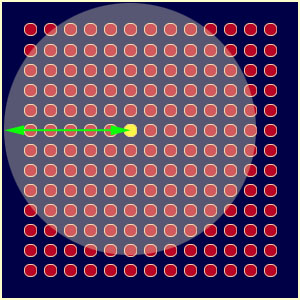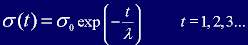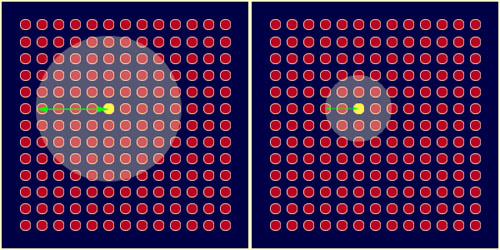Determining the Best Matching Unit's Local Neighbourhood

This is where things start to get more interesting! Each iteration, after the BMU has been determined, the next step is to calculate which of the other nodes are within the BMU's neighbourhood. All these nodes will have their weight vectors altered in the next step. So how do we do that? Well it's not too difficult... first you calculate what the radius of the neighbourhood should be and then it's a simple application of good ol' Pythagoras to determine if each node is within the radial distance or not. Figure 4 shows an example of the size of a typical neighbourhood close to the commencement of training.Figure 4

The BMU's neighbourhood.

You can see that the neighbourhood shown above is centered around the BMU (coloured yellow) and encompasses most of the other nodes. The green arrow shows the radius

A unique feature of the Kohonen learning algorithm is that the area of the neighbourhood shrinks over time. This is accomplished by making the radius of the neighbourhood shrink over time (that was hard to work out eh? ;0) ). To do this I use the exponential decay function:equation 2

where the Greek letter sigma, σ0, denotes the width of the lattice at time t 0 and the Greek letter lambda, λ, denotes a time constant.  t is the current time-step (iteration of the loop). In my code I name the value σ,  m_dMapRadius, and it is equal to σ0 at the commencement of training. This is how I calculate σ0.

The value of λ is dependent on σ and the number of iterations chosen for the algorithm to run. I have named λ, m_dTimeConstant and it is calculated by the line:

m_iNumIterations is the number of iterations the learning algorithm will perform. This value is set by the user in 'constants.h'.

We can use m_dTimeConstant and m_dMapRadius to calculate the neighbourhood radius for each iteration of the algorithm using Equation 2. The line that does this in the source code can be found inside Csom::Epoch and it looks like this:

Figure 5 shows how the neighbourhood in Figure 4 decreases over time (the figure is drawn assuming the neighbourhood remains centered on the same node, in practice the BMU will move around according to the input vector being presented to the network) .Figure 5

Over time the neighbourhood will shrink to the size of just one node... the BMU.

Now we know the radius, it's a simple matter to iterate through all the nodes in the lattice to determine if they lay within the radius or not. If a node is found to be within the neighbourhood then its weight vector is adjusted as follows...

1   2   3   4   5   Home